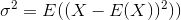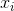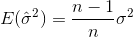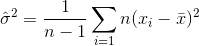# Desvio padrão python x matlab

Adaptado do original: Why does numpy std() give a different result to matlab std()?

PERGUNTA

I try to convert matlab code to numpy and figured out that numpy has a different result with the std function.

in matlab

``````std([1,3,4,6])
ans =  2.0817``````

in numpy

``````np.std([1,3,4,6])
1.8027756377319946``````

Is this normal? And how should I handle this?

RESPOSTA

The NumPy function `np.std` takes an optional parameter `ddof`: “Delta Degrees of Freedom”. By default, this is `0`. Set it to `1` to get the MATLAB result:

``````>>> np.std([1,3,4,6], ddof=1)
2.0816659994661326``````

To add a little more context, in the calculation of the variance (of which the standard deviation is the square root) we typically divide by the number of values we have.

But if we select a random sample of `N` elements from a larger distribution and calculate the variance, division by `N` can lead to an underestimate of the actual variance. To fix this, we can lower the number we divide by (the degrees of freedom) to a number less than `N` (usually `N-1`). The `ddof` parameter allows us change the divisor by the amount we specify.

Unless told otherwise, NumPy will calculate the biased estimator for the variance (`ddof=0`, dividing by `N`). This is what you want if you are working with the entire distribution (and not a subset of values which have been randomly picked from a larger distribution). If the `ddof`parameter is given, NumPy divides by `N - ddof` instead.

The default behaviour of MATLAB’s `std` is to correct the bias for sample variance by dividing by `N-1`. This gets rid of some of (but probably not all of) of the bias in the standard deviation. This is likely to be what you want if you’re using the function on a random sample of a larger distribution.

The nice answer by @hbaderts gives further mathematical details.

The standard deviation is the square root of the variance. The variance of a random variable `X` is defined asAn estimator for the variance would therefore bewheredenotes the sample mean. For randomly selected, it can be shown that this estimator does not converge to the real variance, but toIf you randomly select samples and estimate the sample mean and variance, you will have to use a corrected (unbiased) estimatorwhich will converge to. The correction termis also called Bessel’s correction.

Now by default, MATLABs `std` calculates the unbiased estimator with the correction term `n-1`. NumPy however (as @ajcr explained) calculates the biased estimator with no correction term by default. The parameter `ddof` allows to set any correction term `n-ddof`. By setting it to 1 you get the same result as in MATLAB.

Similarly, MATLAB allows to add a second parameter `w`, which specifies the “weighing scheme”. The default, `w=0`, results in the correction term `n-1` (unbiased estimator), while for `w=1`, only n is used as correction term (biased estimator).